# Resultant of Coplanar Forces Notes pdf ppt

## Resultant of Co-planar Forces:

When a number of coplanar forces are acting on a rigid* body, then these forces can be replaced by a single force which has the same effect on the rigid body as that of all the forces acting together, then this single force is known as the resultant of several forces. Hence a single force which can replace a number of forces acting on a rigid body, without causing any change in the external effects on the body, is known as the resultant force.

The resultant of coplanar forces may be determined by the following two methods :

1. Graphical method

2. Analytical method.

The resultant of the following coplanar forces will be determined by the above two methods:

(i) Resultant of collinear coplanar forces

(ii) Resultant of concurrent coplanar forces.

## Resultant of Collinear Coplanar Forces:

Collinear Coplanar forces are those forces which act in the same plane and have a common line of action. The resultant of these forces are obtained by analytical method or graphical method.

## Analytical method:

The resultant is obtained by adding all the forces if they are acting in the same direction. If any one of the forces is acting in the opposite direction, then resultant is obtained by subtracting that force.

Fig. 1.36 shows three collinear coplanar forcesF1, F2 andF3 acting on a rigid body in the same direction. Their resultant R will be sum of these forces.

R = F 1 + F2 + F3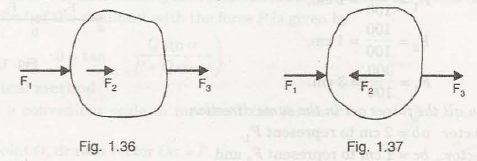If any one of these forces (say force F2 ) is acting in the opposite direction, as shown in

Fig. 1.37, then their resultant will be given by

R =F1 -F2 +F3

## Graphical method:

Some suitable scale is chosen and vectors are drawn to the chosen scale. These vectors are added/ a or subtracted to find the resultant. The resultant of the three collinear forces F1‘ F2 and F3 acting in the same direction will  be obtained by adding all the vectors. In Fig. 1.38, the force F1 = ab to some scale, force F2 =be and force Fa= ed. Then the length ad represents the magnitude of the resultant on the scale chosen.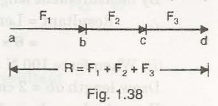The resultant of the forces F1‘ F2 and Fa acting on a body shown in Fig. 1.37 will be obtained by subtracting the vector F2. This resultant is shown in Fig. 1.39, in which the force F1 = ab a to some suitable scale. This force is acting from a to b. The force 1 F2 is taken equal to be on the same scale in opposite direction. This force is acting from b to c. The force Fa is taken equal to cd. This force is acting from c to d. The resultant force is represented in magnitude by ad on the chosen scale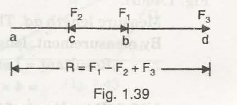## Resultant of Concurrent Coplanar Forces:

Concurrent coplanar forces are those forces which act in the same plane and they intersect or meet at a common point. We will consider the following two cases:

(i) When two forces act at a point

(ii) When more than two forces act at a point.

## (a) Analytical method

when two forces act at a point, their resultant is found by the law of parallelogram of forces. The magnitude of resultant is obtained from equation (1.1) and the direction of resultant with one of the forces is obtained from equation (1.2).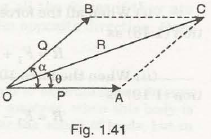Suppose two forces P and Q act at point 0 as shown in Fig. 1.41 and a is the angle between them. Let e is the angle made by the resultant R with the direction of force P ..

Forces P and Q form two sides of a parallelogram and according to the law, the diagonal through the point O gives the resultant R as shown.

The magnitude* of resultant is given by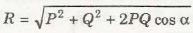The above method of determining the resultant is also known as the cosine law method.

The direction* of the resultant with the force P is given by

θ = tan -1 ( Q sin a / P + Q cos a)

## (b) Graphical method:

(i) Choose a convenient scale to represent the forces P and Q.

(ii) From point 0 , draw a vector Oa = P.

(iii) Now from point 0, draw another vector Ob = Q and at an angle of a as shown in Fig. 1.42.

(iv) Complete the parallelogram by drawing linesa || to Ob and be || to Oa.

(v) Measure the length Oc.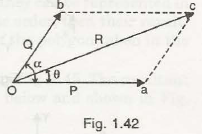Then resultant R will be equal to length Oc x chosen scale.

(vi) Also measure the angle e, which will give the direction of resultant.

The resultant can also be determine graphically by drawing a triangle oac as explained below and shown in Fig. 1.43.

(i) Draw a line oa parallel to P and equal to P.

(ii) From a, draw a vector ac at an angle a with the horizontal and cut ac equal to Q.

(iii) Join oc. Then oc represents the magnitude and direction of resultant R.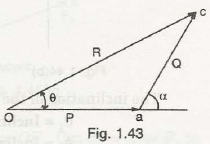Magnitude of resultant R = Length Oc x chosen scale. The direction of resultant is given by angle θ. Hence measure the angle θ.

## (a) Analytical method.

The resultant of three or more forces acting at a point is found analytically by a method which is known as rectangular components methods . According to this method all the forces acting at a point are resolved into horizontal and vertical components and then algebraic summation** of horizontal and vertical components is done separately. The summation of horizontal component is written as ΣH and that of vertical as ΣV. Then resultant R is given by

The angle made by the resultant with horizontal is given by

tan θ = ( ΣV / ΣH)

Let four forces F1 F2, F3 and F4 act at a point 0 as shown in Fig. 1.44.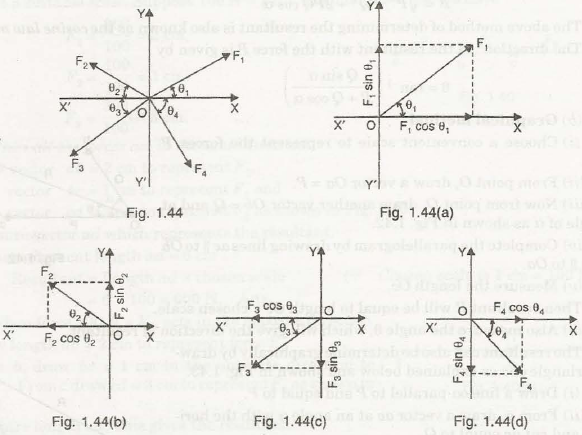The inclination of the forces is indicated with respect to horizontal direction. Let

θ1 =Inclination of force F1 with OX

θ2 = Inclination of force F2 with OX’

θ3 = Inclination of force F3 with OX’

θ4 =Inclination of force F4 with OX.

The force F 1 is resolved into horizontal and vertical components and these components are shown in Fig. 1.44(a). Similarly, Figs. 1.44(b), (c) and (d) show the horizontal and vertical components of forces F2, F3 and F4 respectively. The various horizontal components are:

F1 cos θ1 à  (+)

F2 cos θ 2 à (-)

F3 cos θ 3 à (-)

F4 cos θ4 à (+)

Summation or algebraic sum of horizontal components :

ΣH F1 cos θ1 -F2 cos θ2 -F3 cos θ3 + F4 cos θ4

Summation or algebraic sum of vertical components:

ΣH = F1 sin θ1 + F2 sin θ2 -F3 sin θ3 -F4 sin θ4

And the angle (θ) made by resultant with x-axis is given by tan θ = (ΣH / ΣH)

(b) Graphical method. The resultant of several forces acting at a point is found graphically with the help of the polygon law of forces, which may be stated as

“If a number of coplanar forces are acting at a point such that they can be represented in magnitude and direction by the sides of a polygon taken in the same order, then their resultant is represented in magnitude and direction by the closing side of the polygon taken in the opposite order.

Let the four forces F1, F2 , F 3 and F4 act at a point 0 as shown in Fig. 1.45. The resultant 1s obtained graphically by drawing polygon of forces as explained below and shown in Fig. 1.45(a).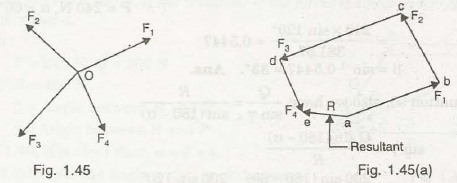(i) Choose a suitable scale to represent the given forces.

(ii) Take any point a. From a, draw vector ab parallel to force OF1. Cut ab =force F 1 to the scale.

(iii) From point b, draw be parallel to OF2. Cut be= force F2:

(iv) From point C, draw cd parallel to OF3. Cut cd =force F3.

(v) From point d, draw de parallel to OF4 Cut de= force F4 .

(vi) Join point a to e. This is the closing side of the polygon. Hence are represents theresultant in magnitude and direction.

Magnitude of resultant R = Length ae x scale.

The resultant is acting from a to e.

### Feedback is important to us.

error: Content is protected !!